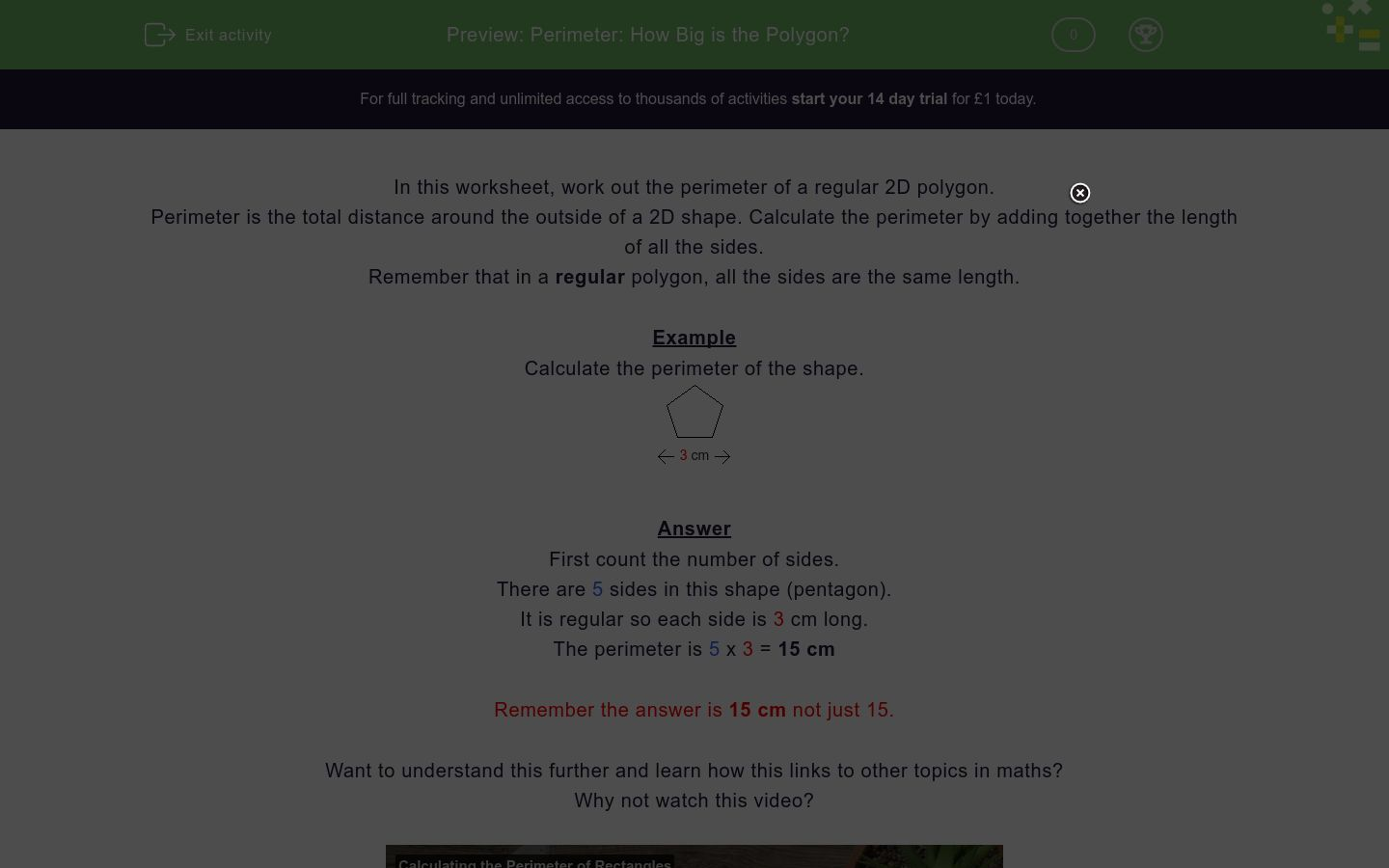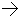# Perimeter: How Big is the Polygon?

In this worksheet, students work out the perimeter of a regular 2D polygon.Key stage:  KS 2

Curriculum topic:   Measurement

Curriculum subtopic:   Measure and Calculate Shapes

Difficulty level:### QUESTION 1 of 10

In this worksheet, work out the perimeter of a regular 2D polygon.

Perimeter is the total distance around the outside of a 2D shape. Calculate the perimeter by adding together the length of all the sides.

Remember that in a regular polygon, all the sides are the same length.

Example

Calculate the perimeter of the shape.3 cmFirst count the number of sides.

There are 5 sides in this shape (pentagon).

It is regular so each side is 3 cm long.

The perimeter is 5 x 3 = 15 cm

Remember the answer is 15 cm not just 15.

Want to understand this further and learn how this links to other topics in maths?
Why not watch this video?

Calculate the perimeter of the shape.10 cmRemember to write the answer in cm.

Calculate the perimeter of the shape.5 cmRemember to write the answer in cm.

Calculate the perimeter of the shape.6 cmRemember to write the answer in cm.

Calculate the perimeter of the shape.10 cmRemember to write the answer in cm.

Calculate the perimeter of the shape.10 cmRemember to write the answer in cm.

Calculate the perimeter of the shape.8 cmRemember to write the answer in cm.

Calculate the perimeter of the shape.6 cmRemember to write the answer in cm.

Calculate the perimeter of the shape.5 cmRemember to write the answer in cm.

Calculate the perimeter of the shape.9 cmRemember to write the answer in cm.

Calculate the perimeter of the shape.7 cmRemember to write the answer in cm.

• Question 1

Calculate the perimeter of the shape.10 cmRemember to write the answer in cm.
70cm
70 cm
EDDIE SAYS
10 x 7 = 70 cm
• Question 2

Calculate the perimeter of the shape.5 cmRemember to write the answer in cm.

25 cm
25cm
EDDIE SAYS
5 x 5 = 25 cm
• Question 3

Calculate the perimeter of the shape.6 cmRemember to write the answer in cm.

48cm
48 cm
EDDIE SAYS
8 x 6 = 48 cm
• Question 4

Calculate the perimeter of the shape.10 cmRemember to write the answer in cm.

80 cm
80cm
EDDIE SAYS
8 x 10 = 80 cm
• Question 5

Calculate the perimeter of the shape.10 cmRemember to write the answer in cm.

100cm
100 cm
EDDIE SAYS
10 x 10 = 100 cm
• Question 6

Calculate the perimeter of the shape.8 cmRemember to write the answer in cm.

72 cm
72cm
EDDIE SAYS
9 x 8 = 72 cm
• Question 7

Calculate the perimeter of the shape.6 cmRemember to write the answer in cm.

48 cm
48cm
EDDIE SAYS
8 x 6 = 48 cm
• Question 8

Calculate the perimeter of the shape.5 cmRemember to write the answer in cm.

40cm
40 cm
EDDIE SAYS
8 x 5 = 40 cm
• Question 9

Calculate the perimeter of the shape.9 cmRemember to write the answer in cm.

81cm
81 cm
EDDIE SAYS
9 x 9 = 81 cm
• Question 10

Calculate the perimeter of the shape.7 cmRemember to write the answer in cm.

49cm
49 cm
EDDIE SAYS
7 x 7 = 49 cm
---- OR ----

Sign up for a £1 trial so you can track and measure your child's progress on this activity.

### What is EdPlace?

We're your National Curriculum aligned online education content provider helping each child succeed in English, maths and science from year 1 to GCSE. With an EdPlace account you’ll be able to track and measure progress, helping each child achieve their best. We build confidence and attainment by personalising each child’s learning at a level that suits them.

Get started